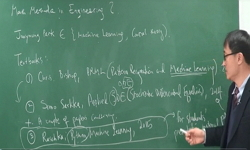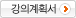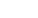### 주메뉴

### 응용수학2

• 고려대학교
• 박주영• 주제분류
자연과학 >수학ㆍ물리ㆍ천문ㆍ지리 >수학
• 강의학기
2016년 1학기
• 조회수
8,111
•
강의계획서기계학습(machine learning)에 사용되는 각종 응용수학관련 주제를 다룸
Introduction#### 차시별 강의1.Introduction Course overview, PGMIntroduction Course overview, PGM2.Special topic: AlphaGo AlphaGo algorithmsSpecial topic: AlphaGo AlphaGo algorithms3.Graphical models (ch.8) PGMGraphical models (ch.8) PGM4.Mixture models & EM (ch.9) MoG, EMMixture models & EM (ch.9) MoG, EM5.Approximate inference (ch.10) Mean field approach,…Approximate inference (ch.10) Mean field approach,…6.Approximate inference (ch.10) EP,…Approximate inference (ch.10) EP,…7.Sampling methods (ch.11) Rejection sampling,…Sampling methods (ch.11) Rejection sampling,…8.Continuous latent variables (ch.12) PCA,…Continuous latent variables (ch.12) PCA,…9.Continuous latent variables (ch.12) KPCA,…Continuous latent variables (ch.12) KPCA,…10.Sequential data (ch.13) HMM,…Sequential data (ch.13) HMM,…11.Stochastic differential equations Stochastic process,…Stochastic differential equations Stochastic process,…12.Stochastic differential equations Brownian motion,…Stochastic differential equations Brownian motion,…13.Stochastic differential equations FPK equation,…Stochastic differential equations FPK equation,…#### 연관 자료#### 사용자 의견

강의 평가를 위해서는 로그인 해주세요.

#### 이용방법

• 플래쉬 유형 강의 이용시 필요한 프로그램 [바로가기]
문서 자료 이용시 필요한 프로그램 [바로가기]

※ 강의별로 교수님의 사정에 따라 전체 차시 중 일부 차시만 공개되는 경우가 있으니 양해 부탁드립니다.